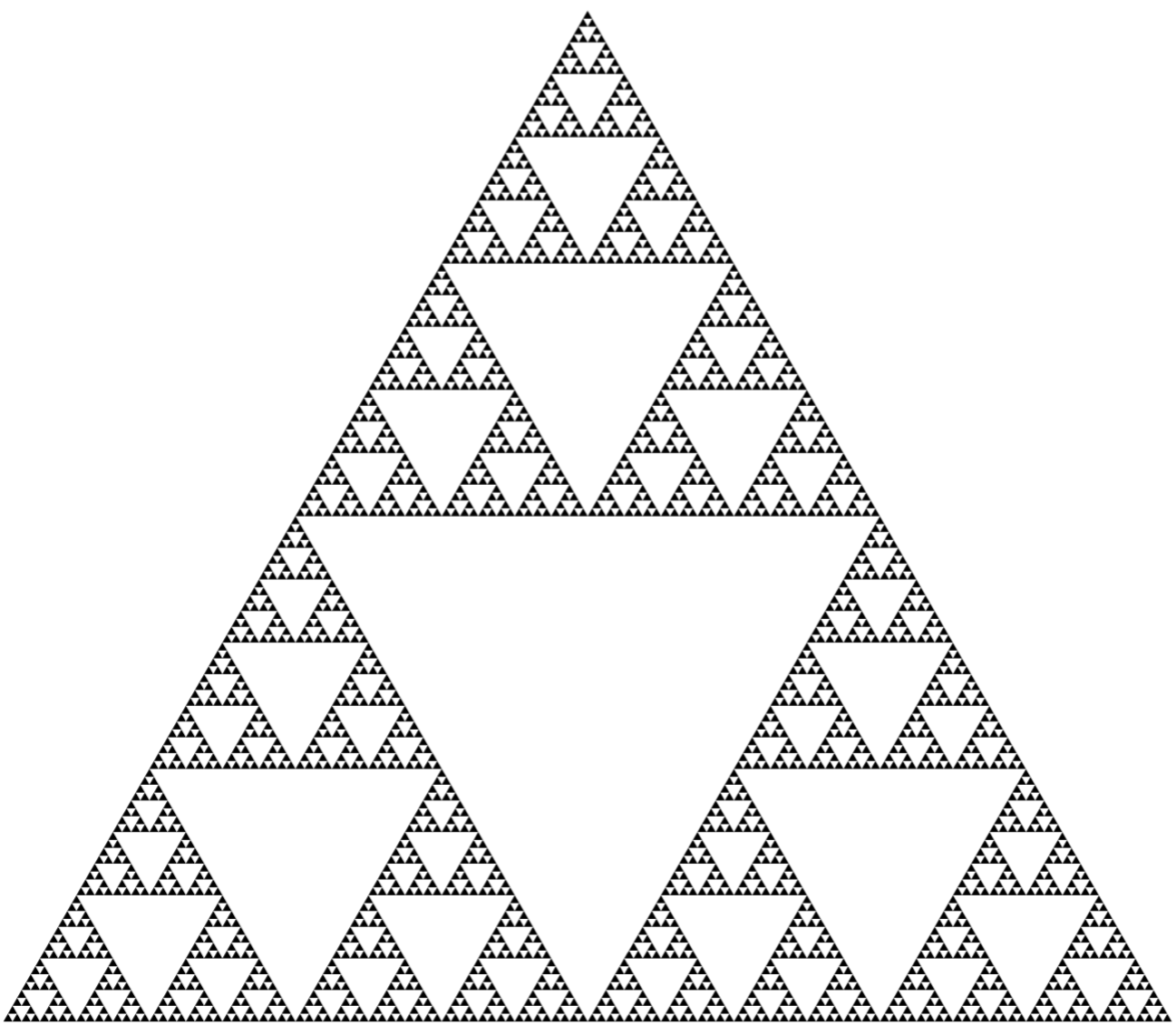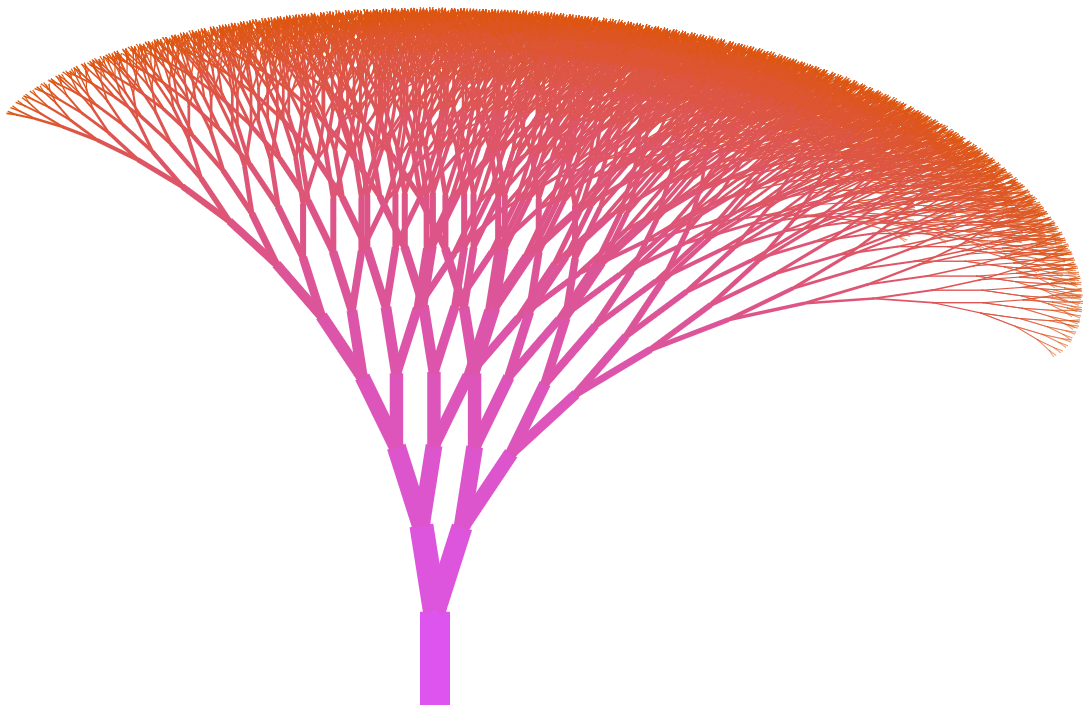# Use similarity to understand sums with infinitely many terms

A series is a sum of infinitely many terms. In some cases the terms have an understandable pattern and we can calculate the number that the sum represents. For example, this is one way to understand repeating decimals like .11111... = 1/9 or .99999.... = 1.Stacked end-to-end, the lengths of these boxes add up to a geometric series 1 + 1/2 + 1/4 + 1/8 + 1/16 + .... You can use similarity to see that the total width is 2.

Using scaling relationships to understand series is a very old idea — at least as old as Archimedes — but is still a cool one. The Wikipedia article on Geometric series has some other nice pictures to explain and think about them.

## Fractal patterns

Fractal patterns give intuitive meaning to some other series, and we can use this together with principles of similarity to calculate these series. Here are four fun examples.

### Heart fractalThe heart fractal is formed by adding and taking away infinitely many heart shapes, each a 1/2 scaling of the previous one.

Since each smaller heart is scaled by a factor of 1/2 from the previous one, each heart area is 1/4 of the previous one. This means the total red area can be described using an infinite sequence of additions and subtractions (a series) as

1 - 1/4 + 1/16 - 1/64 + 1/256 - ...

But we can also use a scaling relationship between the total region remaining (red) and the total region subtracted (white), and the fact that they combine to form the largest heart shape. This gives two equations that these two values satisfy, and so we can solve to deduce the value of the series!

### Sierpinski triangleThe Sierpinski triangle is formed by starting with an equilateral traingle and removing a smaller equilateral triangle from the middle, then smaller ones from each of the remaining 3 triangles, and so on.

We can use the same idea here: Write an expression for the total area removed as a series (the white area in the picture). Use a scaling relationship between the total white area and the portion in each corner of the large triangle to write an equation that the white area satisfies. Use this do deduce the value of the series! Confirm your answer by recalling the value of a geometric series.

### Tree fractalThe tree fractal is made by starting with the base rectangle, then adding two scaled copies on top of it, then two scaled copies of those on top of each one, and so on. The angles at which the branches are attached determine how wide it spreads, and give it a gentle tilt.

In the picture here, each branch is 2/3 the length of the one before it. The total length from the base to a point on the top of the tree is the series formed by adding the lengths of each branch along the way. This forms another geometric series!

### More squares!

This picture shows how to calculate the value of the series

1 + 2/4 + 3/16 + 4/64 + 5/256 + 6/1024 + ...The total area here can be described as a series, but not a geometric series.

This isn't a geometric series, even though it's related to geometry, because the terms aren't simply powers of a single base number. But the picture shows a scaling relationship that can still help us understand its value. If you scale this picture by a factor of 1/2 and remove the scaled-down part, you will be left with some area which is described by a geometric series! Find the value of that geometric series, and then use arithmetic to deduce the value of the original one.This area does form a geometric series!

## Warnings!!

The geometry of these pictures makes the related series easy to calculate, but there are other series which are not so easy to work with. The commutative and associative properties don't always hold for values of series (but they do for the ones related to geometry as above)! And not every series you write down has a well-defined numerical value. For example, the Harmonic series is interesting, but does not converge to a specific number.The content of nilesjohnson.net is licensed under CC BY-NC 4.0.